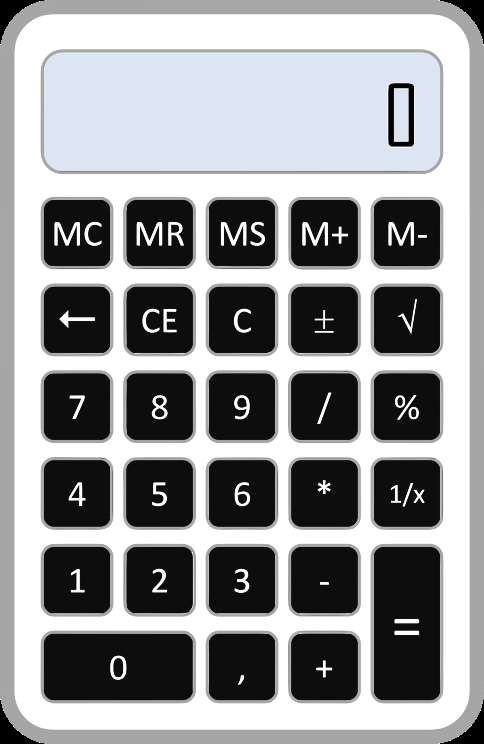# Exponent Calculator

Instructions: Use this exponent calculator to compute any operation involving exponents, showing all the steps. Please type a valid expression involving exponentials in the form box below.Enter the exponent expression you want to calculate (Ex: 2^3 * 2^(1/2), etc.)

Here is a little more about exponent calculator with steps: This calculator will allow compute an simplify numeric expressions that involve exponents. You need to provide a valid expression that involves exponents. For example, you can provide something like '2^(1/2) *2^(1/3)'.

All you need to do is to provide a valid numeric expression that involves exponent, and then click on "Calculate".

Typically, expressions involving exponents will allow some kind of simplifications when terms with exponents are being multiplied.## How to simplify or calculate exponents?

Exponents commonly appears in Algebra, and naturally so in many contexts. Exponents are easy to deal provided that certain structures are present. In order for simplifications to be easy, you will have to have multiplications and the same base, but that is not the only way.

## What are the rules for operating exponents?

We could make this list more compact, but these are main exponent rules that will help you simplify expressions

• Rule 1: $$a^m \cdot a^n = a^{m+n}$$
• Rule 2: $$\displaystyle \frac{a^m}{a^n} = \displaystyle a^{m-n}$$
• Rule 3: $${a^{m}}^n = a^{mn}$$
• Rule 4: $$(ab)^m = a^m b^m$$
• Rule 5: $$\displaystyle \left(\frac{a}{b}\right)^m = \displaystyle \frac{a^m}{b^m}$$
• Rule 6: $$a^{-m} = \displaystyle \frac{1}{a^m}$$
• Rule 7: $$a^0 = 1$$
• Rule 8: $$a^{\frac{m}{n}} = \displaystyle \sqrt[n]{a^m}$$

Some rules are redundant in that list, and we could derive these from a smaller set of rules, but that is not our main goal now.

## How to operate exponents?

It seems that I am not giving an answer to that, but the answer is: use the rules presented above. There is no one correct way to go about exponential expressions, other than respecting the operations order, and start simplifying easy, simple terms first.

Then, based on the structure of what you have, you can collapse terms with exponents, dependent on whether they have the same base, or the same exponent, or any other structure that can make use of the rules presented above..

## Why is it important to compute exponents?

Exponents are a natural occurrence in algebraic terms, and knowing how to handle them and mostly reduce those exponents when possible can prove a very valuable skill in your arsenal.

Always remember to start with the easier parts of the expressions, and try to group things using the above rule, looking for easier intermediate things to simplify.

## Is a square root calculator the same as an exponent calculator?

A square root calculator is a type of exponent calculator. Indeed, when you have a basic square root like $$\sqrt x$$, this is actually represented by an exponent, because

$\sqrt x = x^{\frac{1}{2}}$

Therefore, there is an exponent involved and the rules of exponents will apply. Of course, some people will argue that the function itself it is not an exponential function, which is correct, because in the case of $$\sqrt x$$, the argument of the function is the base and not the exponent.### Example: Calculating an exponent

Calculate the following: $$2^3 + 3^2$$

Solution: We get

$2^3 + 3^2 = 8 + 9 = 17$

which concludes the calculation.

### Example: Another exponent calculation

Calculate $$\left(\frac{2}{3} \right)^{2} \times 6^2$$.

Solution: We find that

$\left(\frac{2}{3} \right)^{2} \times 6^2 = \frac{4}{9} \times 36 = 16$

which concludes the calculation.

## More Algebra calculators

Exponents are not the only operations of importance in Algebra, although they are very prevalent in most algebraic expressions you encounter. Fractions are also important, and you can use this fraction reduction calculator, to reduce a given fraction, or even better to use this fraction calculator to handle any fraction operation. Also related to fractions, you can try to convert fraction to percentage or fraction to decimal.